## 1. 目标检测问题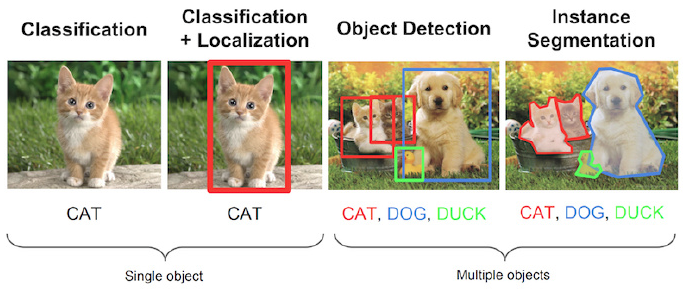## 2. 目标检测模型的评测

### 2.2. 关于 GroundTruth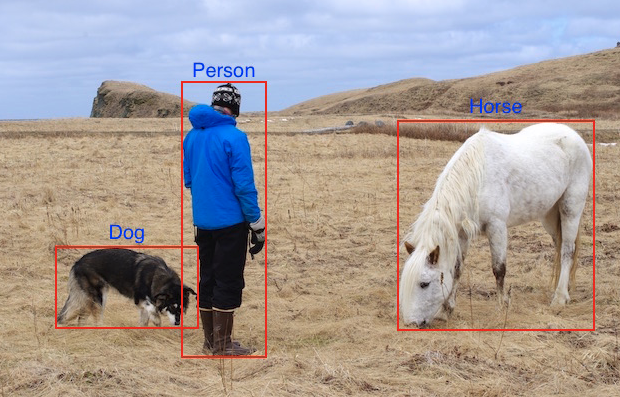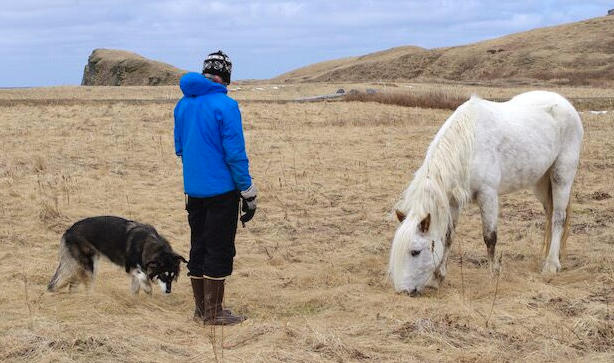ClassX coordinateY coordinateBox WidthBox Height
Dog100600150100
Horse700300200250
Person400400100500

## 3. mAP 计算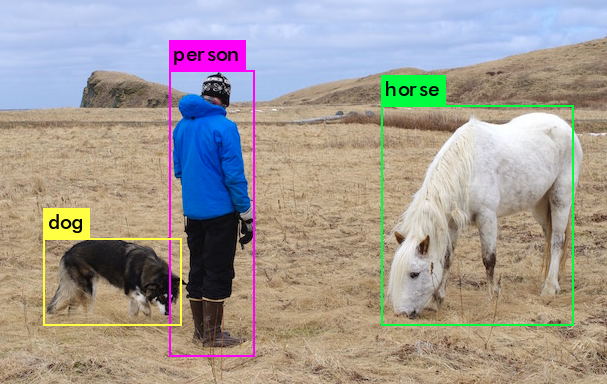### 3.1. IoU

IoU 是预测框与 GT 框的交集与并集的比值. 其是在 20 世纪初 Paul Jaccard 首先提出的.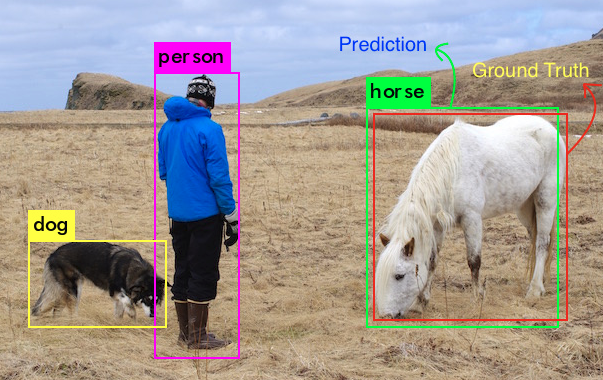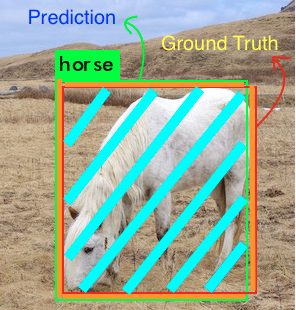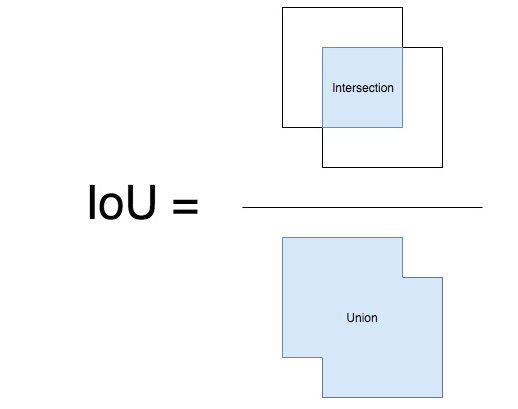### 3.2. 判断正确的检测结果并计算 Precision 和 Recall

$$Precision = \frac{TP}{TP + FP}$$

$$Precision_C = \frac{N(TP)_C}{N(Total)_C}$$

$$Recall = \frac{TP}{TP + FN}$$

### 3.3. 计算 mAP

mAP(Mean Average Precision) 具有不同的定义，其通常用于信息检索(Information Retrieval) 和目标检测领域. 但，其计算方式各不相同. 这里只是介绍目标检测中的 mAP 计算.

IoU 是一种简单的几何度量，很容易标准化. 比如 PASCAL VOC 中采用的 IoU 阈值为 0.5，在 COCO 竞赛中以 0.05 到 0.95 的多个 IoU 阈值计算 mAP.

For a given task and class, the precision/recall curve is computed from a method’s ranked output.

Recall is defined as the proportion of all positive examples ranked above a given rank.

Precision is the proportion of all examples above that rank which are from the positive class.

The AP summarises the shape of the precision/recall curve, and is defined as the mean precision at a set of eleven equally spaced recall levels [0,0.1,…,1]:

The precision at each recall level r is interpolated by taking the maximum precision measured for a method for which the corresponding recall exceeds r:

$$AP_C = \frac{\sum Precision_C}{N(TotalImages)_C}$$

$$mAP = \frac{\sum AP_C}{N(Classes)}$$

## 4. mAP 一些点

 - mAP 一般是对整个数据集进行计算的.

 - 尽管很难简单的评测模型输出的绝对量化，但 mAP 确实是一种相对较好的度量指标. 当对于公开数据集计算 mAP 时，其能够简单有效的对比不同目标检测算法的性能.

 - 取决于训练数据集中的物体类别，Average Precision 值可能对于各类之间变化较大，如，对于质量好的物体类别的训练数据，AP 较高；而质量差的物体类别的训练数据，AP 可能非常低. 因此，模型的 mAP 可能比较一般，但对于某些类别的物体检测可能很好，而对于某些类别的物体检测可能很差. 故，分析模型性能时，分别对每个类别的 AP 进行观察是很有帮助的，其可以作为添加更多训练样本的指示器.

## 5. voc_eval.py

Detectron 中关于 VOC 数据集的 mAP 计算 - voc_eval.py

"""Python implementation of the PASCAL VOC devkit's AP evaluation code."""

import cPickle
import logging
import numpy as np
import os
import xml.etree.ElementTree as ET

logger = logging.getLogger(__name__)

def parse_rec(filename):
"""Parse a PASCAL VOC xml file."""
tree = ET.parse(filename)
objects = []
for obj in tree.findall('object'):
obj_struct = {}
obj_struct['name'] = obj.find('name').text
obj_struct['pose'] = obj.find('pose').text
obj_struct['truncated'] = int(obj.find('truncated').text)
obj_struct['difficult'] = int(obj.find('difficult').text)
bbox = obj.find('bndbox')
obj_struct['bbox'] = [int(bbox.find('xmin').text),
int(bbox.find('ymin').text),
int(bbox.find('xmax').text),
int(bbox.find('ymax').text)]
objects.append(obj_struct)

return objects

def voc_ap(rec, prec, use_07_metric=False):
"""
给定 precision 和 recall，计算 VO AP.
Compute VOC AP given precision and recall.
If use_07_metric is true, uses the VOC 07 11-point method (default:False).
"""
if use_07_metric: # VOC 2007 采用的方法
# 11 point metric
ap = 0.
for t in np.arange(0., 1.1, 0.1):
if np.sum(rec >= t) == 0:
p = 0
else:
p = np.max(prec[rec >= t])
ap = ap + p / 11.
else: # 新方法
# correct AP calculation
# first append sentinel values at the end
mrec = np.concatenate(([0.], rec, [1.]))
mpre = np.concatenate(([0.], prec, [0.]))

# compute the precision envelope
for i in range(mpre.size - 1, 0, -1):
mpre[i - 1] = np.maximum(mpre[i - 1], mpre[i])

# to calculate area under PR curve, look for points
# where X axis (recall) changes value
i = np.where(mrec[1:] != mrec[:-1])

# and sum (\Delta recall) * prec
ap = np.sum((mrec[i + 1] - mrec[i]) * mpre[i + 1])
return ap

def voc_eval(detpath,
annopath,
imagesetfile,
classname,
cachedir,
ovthresh=0.5,
use_07_metric=False):
"""rec, prec, ap = voc_eval(detpath,
annopath,
imagesetfile,
classname,
[ovthresh],
[use_07_metric])

Top level function that does the PASCAL VOC evaluation.

detpath: Path to detections
detpath.format(classname) should produce the detection results file.
annopath: Path to annotations
annopath.format(imagename) should be the xml annotations file.
imagesetfile: Text file containing the list of images, one image per line.
classname: Category name (duh)
cachedir: Directory for caching the annotations
[ovthresh]: Overlap threshold (default = 0.5)
[use_07_metric]: Whether to use VOC07's 11 point AP computation
(default False)
"""
# assumes detections are in detpath.format(classname)
# assumes annotations are in annopath.format(imagename)
# assumes imagesetfile is a text file with each line an image name
# cachedir caches the annotations in a pickle file

# GT
if not os.path.isdir(cachedir):
os.mkdir(cachedir)
imageset = os.path.splitext(os.path.basename(imagesetfile))
cachefile = os.path.join(cachedir, imageset + '_annots.pkl')
with open(imagesetfile, 'r') as f:
imagenames = [x.strip() for x in lines]

if not os.path.isfile(cachefile):
recs = {}
for i, imagename in enumerate(imagenames):
recs[imagename] = parse_rec(annopath.format(imagename))
if i % 100 == 0:
logger.info(
i + 1, len(imagenames)))
# save
logger.info('Saving cached annotations to {:s}'.format(cachefile))
with open(cachefile, 'w') as f:
cPickle.dump(recs, f)
else:
with open(cachefile, 'r') as f:

# extract gt objects for this class
class_recs = {}
npos = 0
for imagename in imagenames:
R = [obj for obj in recs[imagename] if obj['name'] == classname]
bbox = np.array([x['bbox'] for x in R])
difficult = np.array([x['difficult'] for x in R]).astype(np.bool)
det = [False] * len(R)
npos = npos + sum(~difficult)
class_recs[imagename] = {'bbox': bbox,
'difficult': difficult,
'det': det}

# 预测结果
detfile = detpath.format(classname)
with open(detfile, 'r') as f:

splitlines = [x.strip().split(' ') for x in lines]
image_ids = [x for x in splitlines]
confidence = np.array([float(x) for x in splitlines])
BB = np.array([[float(z) for z in x[2:]] for x in splitlines])

####################
# 根据置信度从大到小排序
# sort by confidence
sorted_ind = np.argsort(-confidence)
BB = BB[sorted_ind, :] # 预测矩形框坐标
image_ids = [image_ids[x] for x in sorted_ind] # 预测框对应的图片 id

# 继续计算 TPs 和 FPs
# go down dets and mark TPs and FPs
nd = len(image_ids)
tp = np.zeros(nd)
fp = np.zeros(nd)
for d in range(nd):
R = class_recs[image_ids[d]]
bb = BB[d, :].astype(float)
ovmax = -np.inf
BBGT = R['bbox'].astype(float)

# 计算 IoU
if BBGT.size > 0:
# compute overlaps
# intersection
ixmin = np.maximum(BBGT[:, 0], bb)
iymin = np.maximum(BBGT[:, 1], bb)
ixmax = np.minimum(BBGT[:, 2], bb)
iymax = np.minimum(BBGT[:, 3], bb)
iw = np.maximum(ixmax - ixmin + 1., 0.)
ih = np.maximum(iymax - iymin + 1., 0.)
inters = iw * ih

# union
uni = ((bb - bb + 1.) * (bb - bb + 1.) +
(BBGT[:, 2] - BBGT[:, 0] + 1.) *
(BBGT[:, 3] - BBGT[:, 1] + 1.) - inters)

overlaps = inters / uni
ovmax = np.max(overlaps) # 最大 IoU
jmax = np.argmax(overlaps) # 选择最大的 IoU

if ovmax > ovthresh: # 判断 IoU 阈值
if not R['difficult'][jmax]: # 非 'difficult' 物体
if not R['det'][jmax]:   # 漏检
tp[d] = 1.
R['det'][jmax] = 1   # 标记漏检物体
else:
fp[d] = 1.
else:
fp[d] = 1.

# 计算 precision recall
fp = np.cumsum(fp)
tp = np.cumsum(tp)
rec = tp / float(npos)
# 避免出现 0 为分母，以防第一个检测结果匹配了不同的 GT
# avoid divide by zero in case the first detection matches a difficult
# ground truth
prec = tp / np.maximum(tp + fp, np.finfo(np.float64).eps)
ap = voc_ap(rec, prec, use_07_metric)

return rec, prec, ap
Last modification：May 10th, 2019 at 10:52 am